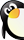### 2014年青岛版小学数学一年级上册期末试题（缺答案）• 需消耗0金币
• 0个贡献点
• 0个黄金点

• 资源类别 试题
资源子类 期末测试
• 教材版本 青岛版
所属学科 小学数学
• 适用年级 一年级
适用地区 全国通用
• 文件大小 909 K
上传用户 rongerkl
• 更新时间 2016/1/11 16:05:38
下载统计 今日0 总计66
• 评论(0) 发表评论 报错(0) 我要报错 收藏
2
2
2014-2015学年第一学期末一年级教学质量检测

 题号 一 二 三 四 五 六 总分 得分

19-7=           4+13=         3+12=           8+7=
5+5=            9+9=           16-9=           5+9=
3+4+6=          7+4+9=         19-8-7=
5+7-3=          13-5+10=       18-6-7=

1、个位上是8，十位上是1，这个数读作（         ）。
2、5个一和1个十合起来是（        ）。
3、16是一个（       ）位数，高位上的数表示1个（       ）。
• 暂时没有相关评论